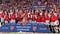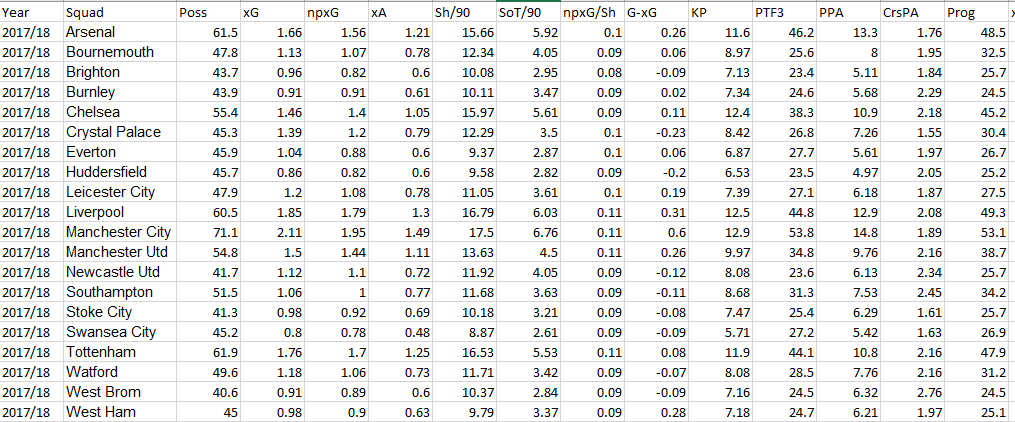# Learning Machines With Me.

A series where I attempt to not only understand, myself, but also do fun things with machine learning — with strangers on the Internet.You are Bart and I am Lisa. Or the other way works as well. We’re both doing some cool stuff.

This is a series where I attempt to do cool things, practical or not, to learn the concepts of machine learning. While you need to understand the math behind the machine-learning, in this series — I will be doing the hard work for you. I will prepare a Google Colab Notebook and provide you a dataset to practice and run the models with.

All you have to do is learn some of the basic level things that are occurring and then, download the dataset and simply run the code! After that, feel free to play around with the code as you see fit and try to make a better model!

Some pre-requisites: Have to know *basic* Python. That’s it!

Let’s get straight to it.

Regression is a concept that most people most intuitively grasp about machine learning. You’ve probably heard of it and if you paid attention in math class, you probably did some rudimentary version of it.

Let’s go through a simple example of what regression attempts to do with some graphs!

Let’s say you wanted to investigate whether passing short passes tells us anything about how a player will pass medium passes. In essence, we are trying to establish a relationship.

Here is a graph of those two variables with the independent variable (short passes attempted) on the x-axis and the dependent variable (medium passes attempted). There seems to be a good correlation — we see that as short passes attempted increases, the medium passes attempted increases.

But let’s say we get a player who is not on the graph — for example, let’s say a player attempts 65 short passes. How many medium passes do we expect him to attempt?

There’s no point on the graph that can help us here — So what do we do?

We can run a regression — this will attempt to correlate these two variables in some sort of relationship that can help us predict any new inputs.

We have two variables so we can do the simplest regression — linear regression. Let’s see how that looks like:

We also get an equation along with something called an R-Squared value. Let’s have a closer look at those:

We have an equation that gets the short passes attempted and converts them to the medium passes attempted with a coefficient (0.654) and an intercept value (6.68).

Ok, this is good but how can we even trust this equation? How much trust should we give?

Well, that’s where the R-Squared value comes which allows us to see how good of a fit the formula was to the dataset. With this value, the closer to 1, the better you can trust the regression formula. In this case, we have a value of 0.35 — not trustworthy at all.

Let’s move onto some final things before we get to actually putting this to code.

There are various types of regressions that you can utilize and each have their requirements, pros, and cons. Here are some:

• Linear Regression
• Multiple Linear Regression
• Polynomial Linear Regression
• Support Vector Regression
• Decision Tree Regression
• Random Forest Regression

For today’s practice, we want to be able to build a regression model that allows us to predict the position of a team.Can we predict where this Arsenal team would stand based on metrics alone?

We will have a lot of variables since there’s a lot of things that go into play to determine a position. Now, you can research the other types of regression and apply them but for this, we’ll be applying random forest regression.

Random forest regression is a type of regression that takes/combines multiple models which makes it a stable and better regression model.

Step 1) Pick how many “trees” we want in our “forest” (How many different regression models we want)

Step 2) We tell the regression to pick K random points from our dataset.

Step 3) Then on those K random points, we run a Decision Tree Regression in the trees we picked in Step 1. We don’t need to worry about the details of what occurs in this step (although you can research it)

Step 4) For predicting a new data input, we make our “trees” in our “forest” predict values for this input and then we take the average of those predictions and spit that out as the final prediction of our regression.

With that out of the way, let’s go to the dataset and code!

# Building Our Regression Model

I have compiled data for EPL Teams from 2021/20–2017/18 from FBRef with variables that detail how a team plays, generally, in attack/defense/passing. This is a snapshot of the dataset:Oh look, the first and last time, Arsenal will be first!

Here are some instructions for running this:

• Download the dataset and store it somewhere (remember where you store this!)
• Make a copy of the Notebook so that you can edit and import the data!
• Click this Folder option:
• Here, find the dataset on your computer/laptop and upload it. (Note: this dataset will only exist for this Notebook while you have the Notebook open. If you close the Notebook and restart it, you will have to reupload the dataset again!)
• Now, you can either run all the cells at once OR run it one by one if you make some changes.
• Clicking Run-All runs all cells. Clicking the brackets ( a play button will appear ) will only run that cell.

At the end of the notebook, you’ll see the regression working on the testing set with its predictions versus what actually happened. See what happens there! You’ve just created your first machine learning model (in this series at least)!

# Some Interactivity:

Now, this series is meant to be interactive so how do you make your own models/improve/change things?

Changing/implementing these features/functions will result in different R² numbers at the end which you can then determine to see whether your change made the model better or worse!

One thing is to change the number of trees in the forest:

`from sklearn.ensemble import RandomForestRegressorregressor = RandomForestRegressor(n_estimators= INSERT NUMBER OF TREES YOU WANT, random_state = 0)regressor.fit(X_train,y_train)`

Another thing is to see how sizes of training sets/testing set changes models:

`from sklearn.model_selection import train_test_splitX_train, X_test, y_train, y_test = train_test_split(X, results, test_size = INSERT A NUMBER BETWEEN 0 AND 1)`

What if you want to run our good ol’ linear regression model on this? (Technically, you won’t be running a linear regression model but rather you’ll be doing a multiple linear regression model since we have more than 2 variables)

`from sklearn.linear_model import LinearRegressionregressor = LinearRegression()regressor.fit(X_train, y_train)`

Please feel free to reach out to me on Twitter if you have any questions. Leave any comments on how to improve!

Writer. Aspiring Computer & Data Scientist. “We’re made of star stuff. We are a way for the cosmos to know itself “ — Carl Sagan

Writer. Aspiring Computer & Data Scientist. “We’re made of star stuff. We are a way for the cosmos to know itself “ — Carl Sagan